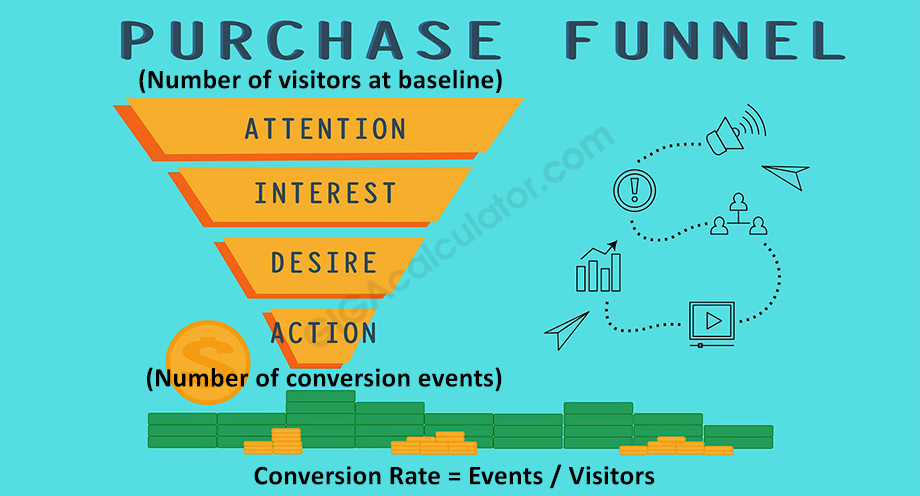# Conversion Rate Calculator

Use this calculator to easily calculate the conversion rate or event rate of a given process: marketing or sales conversions, conversion of visitors to customers, and other types of website conversion rates. Conversion rate formula.

### Calculation results

2% Conversion Rate (%) Conversion Rate
Share calculator:

Embed this tool:
get code

## What is Conversion Rate?

Conversion rate is a ratio that evaluates what proportion of a given population performs a given action. For example, the conversion rate of a sales funnel shows you what proportion of the people who were entered into the funnel made a purchase. In a website scenario a conversion rate might be the proportion of users who complete an e-commerce transaction from the whole users on the site, or from a subset of users. Other conversion rates commonly used in UX and Conversion Rate Optimization are product-to-cart, cart-to-checkout, checkout-to-purchase conversion rate. In advertising a commonly used conversion rate is the CTR (Click-Through Rate) which measures the proportion of ad views that resulted in an ad click and is a primary engagement measure. The conversion rate is a ratio.

As you can see, a conversion rate can be calculated for any action about which you are interested to know what percentage of the total people who could perform the action ended up performing it. Thus, the labels for the inputs of our conversion rate calculator are only a guidance, and you can actually input any two sets of numbers.

Making sure you are calculating your conversion rate against the correct baseline and using correct metrics is important, e.g. when calculating your cart-to-checkout conversion rate (because your are optimize the online shopping cart experience of your users) you should not include in the baseline users who did not see the cart. Similarly, if your site serves both potential customers and already paying customers, as is often the case with subscription services and SaaS, you should exclude your current customers from the baseline, since they cannot convert (unless you consider upgrades, but that is a conversion goal).

## Conversion Rate Formula

Conversion rate is calculated by dividing the number of events of interest by the number of people in the population of interest. It could be ad clicks by ad views, goal conversions by sessions, sales by unique users, etc. This measure is usually expressed in percentages, as it makes comparing the performance of different processes (e.g. via A/B testing), different sources of leads, different segments of the users based on some characteristic, etc.

The CR formula used in our conversion rate calculator is:

Conversion Rate = (Events / Visitors) x 100.

The multiplication by 100 is to convert the simple rate to a percentage. As you can see, the mathematics are very straightforward.

## Practical examples

Below are several practical scenario that involve the need to calculate conversion rate in sales and marketing.

### Example 1: Calculating e-commerce conversion rate.

Let us say you are tasked with calculating the e-commerce conversion rate of a given online shop for the past month. You have data from Google Analytics showing you the number of users that visit the site during the period: 10,000, as well as the number of e-commerce transactions (purchases, sales) that happened: 150. In order to calculate the conversion rate you need to divide 150 by 10,000 to get 0.015, then multiply by 100 to get 1.5% conversion rate.

You should beware when interpreting the result from a calculation as the above since a single user may place more than one order during that time-frime, therefore making interpreting and reporting the number as "percentage of users who purchased" problematic. In order to calculate that conversion rate, you will need to use the number of users who purchased, instead of the number of e-commerce transactions. In case only a few customers per month make more than one purchase this extra effort might not be justified, but in other cases it can be crucial if working with the right numbers and doing a good job is a priority.

### Example 2: Calculating shopping cart conversion rateLet us say you have a goal set that tracks the number of sessions during which a user reaches the checkout of an online store. You are doing some CRO & A/B testing a new shopping cart experience and in order to calculate the sample size for your test of statistical significance you need to estimate the baseline conversion rate for cart to checkout. You are interested in user-level metrics, so you want to know what % of users who see the cart later proceed to the checkout.

To calculate that, you pull up your available web analytics software and see that for the past 3 months the number of users who saw the cart was 300,000 and the number of people who saw the first step of the checkout was 30,000. To calculate the conversion rate you need to divide 30,000 by 300,000, giving you the result of 0.10 or 10% after multiplying by 100. Similar caveats exist as in Example 1, since the same user may reach the cart and the checkout page more than once during the time period, so interpretation of the data may not be trivial. Our calculator would have been most useful in carrying out the math.

## Change of conversion rate over time

Often people want to report on an average increase or decrease (any kind of change) of conversion rate over time. Many think that just taking the arithmetic average is sufficient, but since we are speaking about rate of change of a proportion, you need to use the geometric average to get accurate results. The same logic applies when calculating average annualized returns, for example.

#### Cite this calculator & page

If you'd like to cite this online calculator resource and information as provided on the page, you can use the following citation:
Georgiev G.Z., "Conversion Rate Calculator", [online] Available at: https://www.gigacalculator.com/calculators/conversion-rate-calculator.php URL [Accessed Date: 06 Jun, 2023].